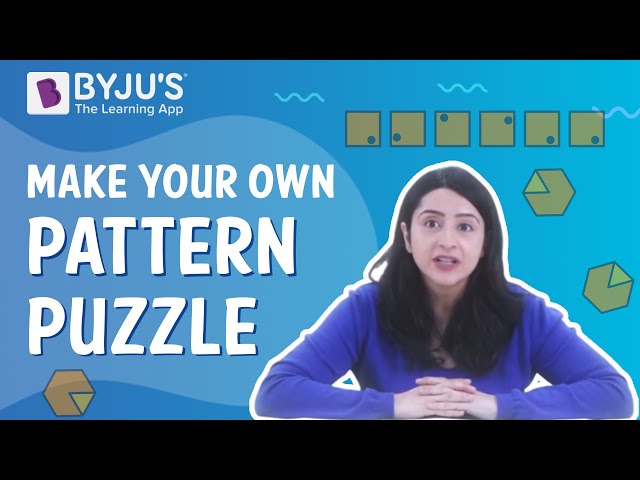Checkout JEE MAINS 2022 Question Paper Analysis : Checkout JEE MAINS 2022 Question Paper Analysis :

# Solving Puzzles Which Involve Numbers And Letters

The number system is one of the most innovative and interesting inventions by human beings. Various tricks and puzzles involving numbers have always made us curious and enhanced our process of thinking. Puzzles were created in order to test the knowledge and thinking ability. In this article, we will enhance our reasoning skills by solving questions on puzzles involving numbers and letters.

There are puzzles in which letters take the place of digits in the arithmetic sum, and we find out which letter represents which digit. It is very much similar to cracking a code. The discussion will stick to problems relating to addition and multiplication.

## Rules for Solving Puzzles

There are two rules which are to be followed for solving the puzzles:

1. In a puzzle each letter stands for a single digit. Each digit should be represented by one letter only.
2. The first digit of a number cannot be zero. For example, we write seventy nine as 79 and not 079.

Illustration 1: Find the value of Q in the following: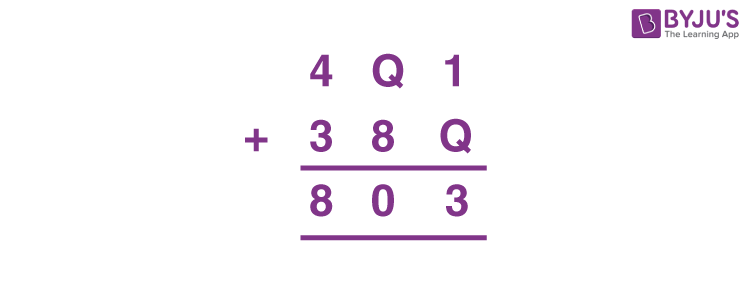Solution:

In column one (starting from right), from Q + 1 we get 3 at the units place.

=> Q + 1 = 3

=> Q = 2

In the middle column,

Q + 8 gives a number such that it has 0 at its units place. So Q = 2. This is verified by the fact that when Q (2) is added to 8 it results in 10 and hence 1 is carried forward. In the third column  4 + 3 + 1(carry) = 8.

Illustration 2: Find the value of A: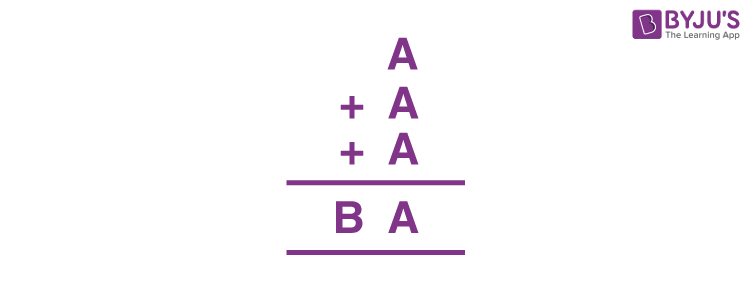Solution:

We have to find the value of A and B. A is any number whose thrice sum with itself is also A. Therefore, the sum of two A’s should be 0. This case is possible only for A = 0 or A = 5.

In this case if A = 0, the entire sum will be 0 and hence B = 0.

But this is not possible as it will lead to A = B

We know that different letters represent different digits. Therefore we take the case in which A = 5.

Hence A + A + A  =  5 + 5 + 5 = 15

So we get B = 1.

Illustration 3: Find the value of B in the following:Solution:

Let the numbers be 10B + 8 and 10B + 3. The product of these numbers will be 644.

=> (10B + 8) (10B + 3) = 644

=> 100B2 + 110B + 24 = 644

=> 100B2 + 110B – 620 = 0

=> 10B2 + 11B – 62 = 0

=> 10B2 – 20B + 31B – 62 = 0

=> 10B (B – 2) + 31 (B – 2) = 0

=> (B – 2) (10B + 31) = 0

=> B = 2                or            B = –

$$\begin{array}{l} \frac {31}{10} \end{array}$$
(this value is discarded as B is an integer)

=> B = 2

Apart from the above type of puzzles, there exists a number of maths puzzles for class 8, class 7 and so on. We can say that  maths puzzles for class 7 involves a certain kind of number pattern. These can be solved using basic arithmetic operations. A few maths puzzles with answers for class 7 are given below:

Puzzle 1: Find the missing number in the puzzle given below.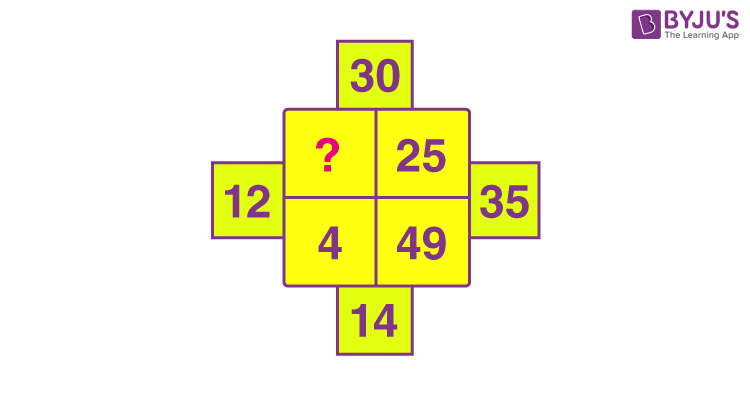Solution:

22 = 4, 72 = 49 and 2 × 7 = 14

72 = 49, 52 = 25 and 7 × 5 = 35

Let the missing number be p2.

Applying the pattern as specified above,

22 = 4 and p × 2 = 12

Thus, p = 6

Hence, the missing number = p2 = 62 = 36

Puzzle 2: What is the missing number?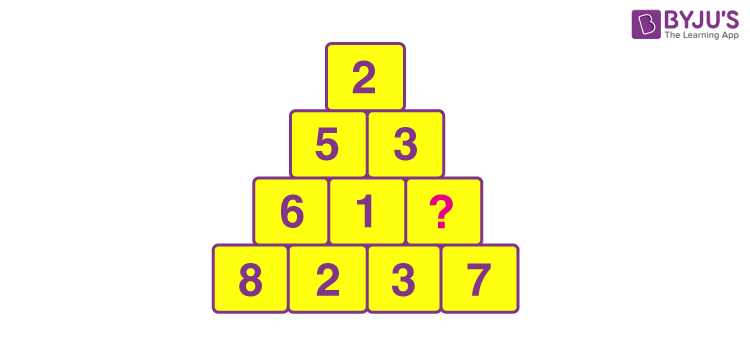Solution:

Let us understand the pattern in the given puzzle.

The numbers in the second row (from the bottom) are the difference of the corresponding two numbers in the bottom row.

That means, 6 = 8 – 2

1 = 3 – 2

7 – 3 = ?

Similarly,

6 – 1 = 5, ? – 1 = 3

And

5 – 3 = 1

From the above, we can say that the missing value is equal to 4.

Also, learn how to make pattern puzzles for class 5 students by watching the video lesson given here.